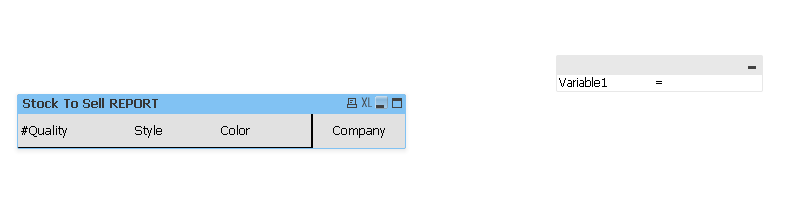# QlikView App Dev

Discussion Board for collaboration related to QlikView App Development.

Announcements
On May 18th at 10AM EDT we will answer your QlikView questions live. REGISTER
cancel
Showing results for
Did you mean:Not applicable

## Input Box Filter Based on Expression

Hey guys,

Quick question

I have a dashboard with a pivot table. The pivot table has many dimensions and one Expression.

The expression is shown in %. I need to create an Input box where a customer can enter a % and it will filter based on the % they inputed..

Any idea's?

Thanks allot1 Solution

Accepted SolutionsMVP

The user will input a number which will be stored in a variable. Lets call the variable vVar and then you will test your expression to see if its above or below it and based on what you want show the value:

If(yourExpression >= \$(vVar), yourExpression)

Does this help? if this is not what you want, can you explain with an example?

12 RepliesMVP

If you want to see rates above user inputs, you can try this

If(YourExpression >= UserInput, YourExpression)

HTH

Best,

SunnyEmployee

Use this type of expression

if(SUM(Value) >= vTextBoxVariable, SUM(Value))Not applicable
Author

The main issue is i don't know how to filter with expression results in an input box. That was my question.MVP

The user will input a number which will be stored in a variable. Lets call the variable vVar and then you will test your expression to see if its above or below it and based on what you want show the value:

If(yourExpression >= \$(vVar), yourExpression)

Does this help? if this is not what you want, can you explain with an example?EmployeeMVP

I would try this:

=If(sum(stkqty)-sum(onorder)-sum(onpick) = \$(vVar), sum(stkqty)-sum(onorder)-sum(onpick))

where vVar will equal 2 based on user input.Not applicable
Author

That worked great.. One more thing when i use this expression when nothing is selected nothing shows up in table how can i change this?MVP

Try this:

=If(Len(Trim(\$(vVar))) = 0, sum(stkqty)-sum(onorder)-sum(onpick), If(sum(stkqty)-sum(onorder)-sum(onpick) = \$(vVar), sum(stkqty)-sum(onorder)-sum(onpick)))Not applicable
Author

mhmm i used this expression and it stopped working altogether.